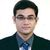Announcements
The way to achieve your own success is the willingness to help somebody else. Go for it!
cancel
Showing results for
Did you mean:MVP

## Like Wise comparision between current month month and last year month

Dear All

Please find attached qlikview – view showing details of current year month and last year month like wise comparision

As follows

 Financial Year  2013 Financial Year  2012 30 March 2013 – working day Working Day 31 th  March is a SUNDAY holiday hence there is  no transcation taken place in this day. Working Day

When we view this report  for march 2013  it is showing data of last year upto 30-March only , But therewas one day sale recored at 31/12/2012 date  Hence growth calculation is going wrong  , Please refer screen short column no 2 is showing 7.6 lacs less sale.

Please find following are the expression for column no 2

(SUM({\$<FinancialYear=,Quarter=,MonthName=,FiscalYear={\$(=max(FiscalYear)-1)},

[Posting Date]={">=\$(=YearStart(max([Posting Date]),-1,4))<=\$(=addmonths(max({\$<FiscalYear={\$(=max(FiscalYear))}>}[Posting Date]),-12))"}>}[Gross Sales Amount])

-

SUM({\$<FinancialYear=,Quarter=,MonthName=,FiscalYear={\$(=max(FiscalYear)-1)},

[Posting Date]={">=\$(=YearStart(max([Posting Date]),-1,4))<=\$(=addmonths(max({\$<FiscalYear={\$(=max(FiscalYear))}>}[Posting Date]),-12))"}>}[Sales Return Amount])

)/100000

Requirement : -

·         If user select March Month Full month completed data should display.

·         If  Today is  2-April-2013   Sales Current Year  Vs   2-April-2012  sales data should display in last year

Kindly advise how to achive the same in said expression.

If the issue is solved please mark the answer with Accept as Solution & like it.
If you want to go quickly, go alone. If you want to go far, go together.
1 Solution

Accepted SolutionsTry this,

SUM({\$<FinancialYear=,Quarter=,MonthName=,FiscalYear={\$(=max(FiscalYear)-1)},

[Posting Date]={">=\$(=YearStart(max([Posting Date]),-1,4))<=\$(=monthend(addyears(max({\$<FiscalYear={\$(=max(FiscalYear))}>}[Posting Date]),-1)))"}>}[Gross Sales Amount])

-

SUM({\$<FinancialYear=,Quarter=,MonthName=,FiscalYear={\$(=max(FiscalYear)-1)},

[Posting Date]={">=\$(=YearStart(max([Posting Date]),-1,4))<=\$(=monthend(addyears(max({\$<FiscalYear={\$(=max(FiscalYear))}>}[Posting Date]),-1)))"}>}[Sales Return Amount])

)/100000

Regards,

Kaushik Solanki

Regards,
Kaushik
9 RepliesHi,

Try this expression.

SUM({\$<FinancialYear=,Quarter=,MonthName=,FiscalYear={\$(=max(FiscalYear)-1)},

-

SUM({\$<FinancialYear=,Quarter=,MonthName=,FiscalYear={\$(=max(FiscalYear)-1)},

)/100000

Regards,

Kaushik Solanki

Regards,
KaushikMVP
Author

Dear Sir,

Thanks

vikas

If the issue is solved please mark the answer with Accept as Solution & like it.
If you want to go quickly, go alone. If you want to go far, go together.Hi,

SUM({\$<FinancialYear=,Quarter=,MonthName=,FiscalYear={\$(=max(FiscalYear)-1)},

[Posting Date]={">=\$(=YearStart(max([Posting Date]),-1,4))<=\$(=addyears(max({\$<FiscalYear={\$(=max(FiscalYear))}>}[Posting Date]),-1))"}>}[Gross Sales Amount])

-

SUM({\$<FinancialYear=,Quarter=,MonthName=,FiscalYear={\$(=max(FiscalYear)-1)},

[Posting Date]={">=\$(=YearStart(max([Posting Date]),-1,4))<=\$(=addyears(max({\$<FiscalYear={\$(=max(FiscalYear))}>}[Posting Date]),-1))"}>}[Sales Return Amount])

)/100000

Regards,

Kaushik Solanki

Regards,
KaushikMVP
Author

Dear Sir

Check the same but Last year posting date taking upto 30/03/2012 only  I want it should be 31/03/2012

since there is no holiday that time.

Please refer screen short g1 for the same.

Vikas

If the issue is solved please mark the answer with Accept as Solution & like it.
If you want to go quickly, go alone. If you want to go far, go together.Try this,

SUM({\$<FinancialYear=,Quarter=,MonthName=,FiscalYear={\$(=max(FiscalYear)-1)},

[Posting Date]={">=\$(=YearStart(max([Posting Date]),-1,4))<=\$(=monthend(addyears(max({\$<FiscalYear={\$(=max(FiscalYear))}>}[Posting Date]),-1)))"}>}[Gross Sales Amount])

-

SUM({\$<FinancialYear=,Quarter=,MonthName=,FiscalYear={\$(=max(FiscalYear)-1)},

[Posting Date]={">=\$(=YearStart(max([Posting Date]),-1,4))<=\$(=monthend(addyears(max({\$<FiscalYear={\$(=max(FiscalYear))}>}[Posting Date]),-1)))"}>}[Sales Return Amount])

)/100000

Regards,

Kaushik Solanki

Regards,
KaushikMVP
Author

Dear Sir,

Thanks this is worked !!!!3 -Apr - 2013  Vs  3-Apr-2012     ( 1 April 2013 to 3  and  1 April 2012  to 3 April 2012  )  will this expression work means when user will see report from 1-April to as on date and same period for last year ( Growth %) purpose will this show correct result ?

Thanks

Vikas

If the issue is solved please mark the answer with Accept as Solution & like it.
If you want to go quickly, go alone. If you want to go far, go together.Hi,

I guess no, this will not work the reason is, we are getting the data between the year start and the monthend of current month of previous year. Meaning if your date is 3-Apr-2013 then the expression will be calculated for 1-Apr-2012 to 30-Apr-2012.

I would suggest you create a master cal seperately where you will have all the days, even tough the transaction has not happened on that day. Then use the master cal to link to your data and then use the master cal to calculate the above expression. In this case use the below expression.

SUM({\$<FinancialYear=,Quarter=,MonthName=,FiscalYear={\$(=max(FiscalYear)-1)},

[Posting Date]={">=\$(=YearStart(max([Posting Date]),-1,4))<=\$(=addyears(max({\$<FiscalYear={\$(=max(FiscalYear))}>}[Posting Date]),-1))"}>}[Gross Sales Amount])

-

SUM({\$<FinancialYear=,Quarter=,MonthName=,FiscalYear={\$(=max(FiscalYear)-1)},

[Posting Date]={">=\$(=YearStart(max([Posting Date]),-1,4))<=\$(=addyears(max({\$<FiscalYear={\$(=max(FiscalYear))}>}[Posting Date]),-1))"}>}[Sales Return Amount])

)/100000

Regards,

Kaushik Solanki

Regards,
KaushikMVP
Author

Dear Sir,

We have master calender already it is base on MIN & MAX date ,

I have done changes in sql script max date of posting date is taken as last day of month whether there is a transaction or not I have tested  in  test scenario I shall test in Live also.

But anyway sir I really appreciate your timely helpThanks

Regards

Vikas

If the issue is solved please mark the answer with Accept as Solution & like it.
If you want to go quickly, go alone. If you want to go far, go together.MVP
AuthorDear Sir

I have done changes in sql max date of posting date taken as last day of month and original expressions giving correct results now. As you suggests for creation of new calender year insted of that I have make chages in sql script only I don't  know this is how much fesible but is this write approch ?